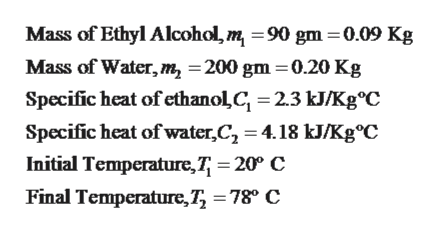# 90.0g of Ethyl alcohol is mixed with 200g of water.  If the mixture is heated from 20 degrees celcius from 78 degrees celcius, what is the energy required to heat the mixture?  ( C ethanol = 2.3 kj/kg C) (CH2O = 4.18 kj/kg C)

Question
14 views

90.0g of Ethyl alcohol is mixed with 200g of water.  If the mixture is heated from 20 degrees celcius from 78 degrees celcius, what is the energy required to heat the mixture?  ( C ethanol = 2.3 kj/kg C) (CH2O = 4.18 kj/kg C)

check_circle

Step 1

Given,help_outlineImage TranscriptioncloseMass of Ethyl Alcohol,m 90 gm 0.09 Kg Mass of Water, m, =200 gm 0.20 Kg Specific heat of ethanolC 2.3 kJ/Kg°C Specific heat of water,C, 4.18 kJ/Kg°C Initial Temperature,7 20° C Final Temperature,7 =78° C fullscreen
Step 2

Energy required to heat is the mixture is equal to sum of heat required by each component of mixture,

Step 3

By substituting the given values the ...

### Want to see the full answer?

See Solution

#### Want to see this answer and more?

Solutions are written by subject experts who are available 24/7. Questions are typically answered within 1 hour.*

See Solution
*Response times may vary by subject and question.
Tagged in

### Other